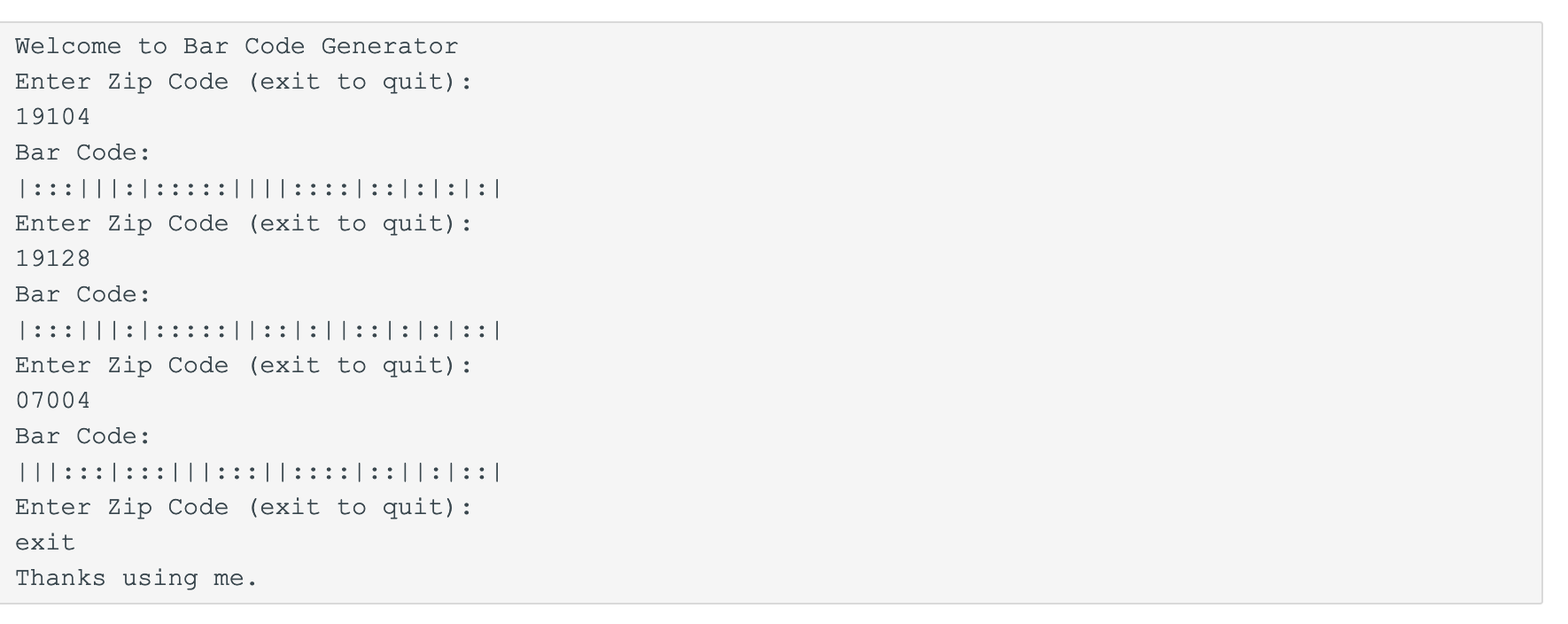Welcome to Bar Code GeneratorEnter Zip Code (exit to quit):19104Bar Code:Enter Zip Code (exit to quit):19128Bar Code:Enter Zip Code (exit to quit):07004Bar Code:Enter Zip Code (exit to quit):exitThanks using me.

Question

Write a program that coverts a zip code to a bar code.

The bar codes use large and small bars. We will use : as a small bar and | as a large bar.

Each digit has its own bar pattern.

• 1 is :::||
• 2 is ::|:|
• 3 is ::||:
• 4 is :|::|
• 5 is :|:|:
• 6 is :||::
• 7 is |:::|
• 8 is |::|:
• 9 is |:|::
• 0 is ||:::

The barcode also includes a check digit. The final digit added to the bar code verifies the previous numbers are correct.

To Determine the checksum:

• Add all the digits together
• Determine what needs to be added to make the total a multiple of 10

The bar code also always starts and ends with a large bar. This is used by the scanner to align the letter.

Drexel's Zip Code is 19104

The check digit is 5. The sum of the digits is 1+9+1+0+4 = 15. To make 15 a multiple of 10, we need to add 5.

The bar code will be |:::|||:|:::::||||::::|::|:|:|:|

The majority of work in this problem is done by digit. It makes sense to store the zip code as a string, not as an integer.

Write the function checksum(zip) that takes the zip code as a string and returns the check digit as a string.

Write a function barcode(zip) that takes a zip code as a string and returns the bar code.

Develop a main program that repeatedly asks the user for zip codes and converts them to bar codes. The program will run until the user enters "exit". Remember to use if name=="main": so that ZyBooks can test your functions.help_outlineImage TranscriptioncloseWelcome to Bar Code Generator Enter Zip Code (exit to quit): 19104 Bar Code: Enter Zip Code (exit to quit): 19128 Bar Code: Enter Zip Code (exit to quit): 07004 Bar Code: Enter Zip Code (exit to quit): exit Thanks using me. fullscreen
Step 1

Since no programming language is mentioned we will use Java to implement solution to this problem. For implementing the code we will make use of String class in Java and Switch statements to generate bar code. Let’s understand first how to define the function checkSum(zip).

Step 2

Following is the implementation of checkSum(zip) function:

public static String checkSum(String zip)

{

int num = Integer.parseInt(zip);          //convert the string variable zip to integer

int temp = num;

int sum =0;

//finding sum of all digits for the given zipcode

while (temp > 0) {

sum = sum + temp % 10;

temp = temp / 10;

}

// returning the check digit for the given zipcode if sum of its digit is not multiple of 10

if (sum%10!=0)

{

int rem = sum%10;

return Integer.toString(10-rem);

}

else

return null;

}

Step 3

We will be using this checkSum() function in our barCode(zip) function to concatenate the check digit to zip code and produce the final bar code. The implementation of barCode(zip) function is as follows:

public static void barCode(String zip)

{

String[] bar = new String[zip.length()+1];      /* declare a string array to store barcode converions

for each digit in zipcode plus one additional string.*/

zip = zip + checkSum(zip);      //concatenate the zip string with the check digit.

//converting each digit in zipcode along with check digit to its barcode notation using switch statement.

for(int i =0; i<zip.length(); i++)

{

switch(Character.getNumericValue(zip.charAt(i)))

{

case 1: bar[i]=":::||";

break;

case 2: bar[i] = "::|:|";

break;

case 3: bar[i]= "::||:";

break;

case 4: bar[i]= ":|::|";

break;

case 5: bar[i]= ":|:|:";

break;

...

Want to see the full answer?

See Solution

Want to see this answer and more?

Our solutions are written by experts, many with advanced degrees, and available 24/7

See Solution
Tagged in

Java